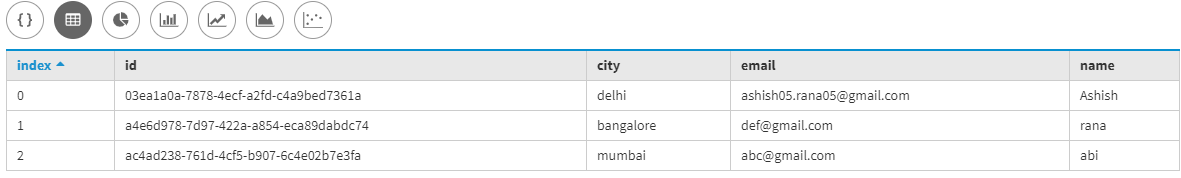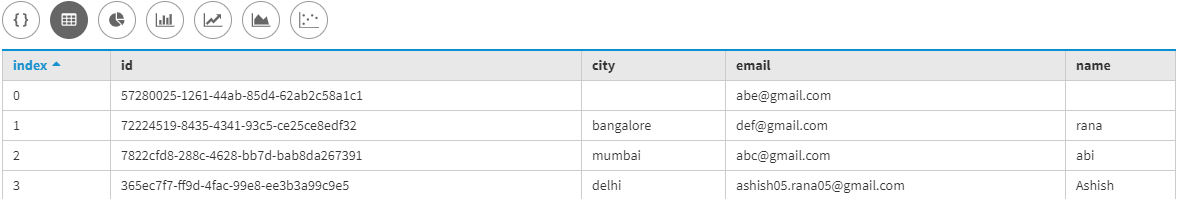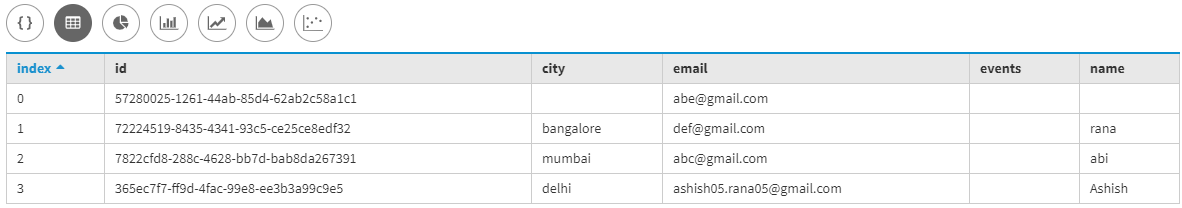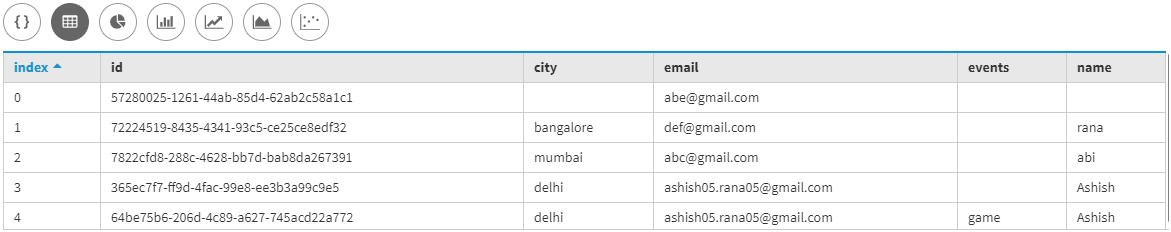# Operations on table in Cassandra

• Last Updated : 31 Dec, 2019

In this article, we will discuss table operations like Create insert truncate drop in Cassandra with some sample exercise. Let’s have a look.

Creating a table – Register:
First, we are going to create a table namely as Register in which we have id, name, email, city are the fields. Let’s have a look.

```Create table Register
(
id uuid primary key,
name text,
email text,
city text
);```

Inserting data into Register table:
After creating a table now, we are going to insert some data into Register table. Let’s have a look.

```Insert into Register (id, name, email, city)
values(uuid(), 'Ashish', 'ashish05.rana05@gmail.com', 'delhi');

Insert into Register (id, name, email, city)
values(uuid(), 'abi', 'abc@gmail.com', 'mumbai');

Insert into Register (id, name, email, city)
values(uuid(), 'rana', 'def@gmail.com', 'bangalore'); ```

Verify the results:
To verify the results using the following CQL query given below. Let’s have a look.

```select *
from Register; ```Describe the table schema:

```describe table Register;

CREATE TABLE cluster1.register (
id uuid,
city text,
email text,
name text,
PRIMARY KEY (id)
AND gc_grace_seconds = 864000
AND bloom_filter_fp_chance = 0.01
AND caching = { 'keys' : 'ALL', 'rows_per_partition' : 'NONE' }
AND comment = ''
AND compaction = { 'class' : 'org.apache.cassandra.db.compaction.SizeTieredCompactionStrategy',
'max_threshold' : 32, 'min_threshold' : 4 }
AND compression = { 'chunk_length_in_kb' : 64,
'class' : 'org.apache.cassandra.io.compress.LZ4Compressor' }
AND default_time_to_live = 0
AND speculative_retry = '99PERCENTILE'
AND min_index_interval = 128
AND max_index_interval = 2048
AND crc_check_chance = 1.0
AND cdc = false
AND memtable_flush_period_in_ms = 0; ```

Updating the table:
To update the table used the following CQL query given below.

```update Register set
email = 'abe@gmail.com'
where id = 57280025-1261-44ab-85d4-62ab2c58a1c1; ```

Verifying after updating the table:
Let’s verify the results by using the following CQL query given below.

```select *
from Register; ```

Output:Alter operation on a table:
To alter the table used the following CQL query given below.

```ALTER table Register

To see the update used the following CQL query given below.

`describe table Register; `

Output:

```CREATE TABLE cluster1.register (
id uuid,
city text,
email text,
events text,
name text,
PRIMARY KEY (id)
AND gc_grace_seconds = 864000
AND bloom_filter_fp_chance = 0.01
AND caching = { 'keys' : 'ALL', 'rows_per_partition' : 'NONE' }
AND comment = ''
AND compaction = { 'class' : 'org.apache.cassandra.db.compaction.SizeTieredCompactionStrategy',
'max_threshold' : 32, 'min_threshold' : 4 }
AND compression = { 'chunk_length_in_kb' : 64,
'class' : 'org.apache.cassandra.io.compress.LZ4Compressor' }
AND default_time_to_live = 0
AND speculative_retry = '99PERCENTILE'
AND min_index_interval = 128
AND max_index_interval = 2048
AND crc_check_chance = 1.0
AND cdc = false
AND memtable_flush_period_in_ms = 0; ```

To verify the newly added column used the following CQL query given below.

```select *
from Register; ```

Output:Inserting data into the new added column:

```Insert into Register (id, name, email, city, events)
values(uuid(), 'Ashish', 'ashish05.rana05@gmail.com', 'delhi', 'game'); ```

To verify inserted data to the newly added column used the following CQL query given below.

```select *
from Register; ```

Output:Deleting data from table Register:
To delete data from table Register used the following CQL query. Let’s have a look.

`truncate Register; `

Delete a table:
To delete table schema and data from table Register used the following CQL query. Let’s have a look.

`drop table Register; `
My Personal Notes arrow_drop_up